Courses
Courses for Kids
Free study material
Free LIVE classes
More

# Circuit Solving - Circuit Theory Problems and Solutions for JEELIVE
Join Vedantu’s FREE Mastercalss

## An Introduction to Circuit Solving

Circuit solving is a cardinal skill required in electrical engineering, which requires a thorough knowledge of active elements and passive elements. It is necessary to understand linear elements individually and laws of circuit theory to solve complicated circuits.

A simple electric circuit consists of a resistor, voltage source, and current flowing through its different branches. A resistor is a passive element that controls the flow of current in the circuit, and the voltage source provides the supply to the circuit. For every circuit, two known electrical quantities and one unknown are given. If the value of voltage source and resistors is given, the value of current is calculated using the Ohm’s ($\Omega$) law.

## Circuit Systems

By definition, a circuit is a closed loop or a circular path in which electrons flow. Not necessarily, a circuit may be closed. There are instances where the current flow can be interpreted or the circuit is incomplete. This is known as an open circuit. Based on the configuration of resistors, the type of sources, and flow of current circuit, systems can be classified as:

1. Open Circuit

2. Closed Circuit

3. DC Circuit

4. AC Circuit

5. Series Circuit

6. Parallel Circuit

7. Series-Parallel Circuit

### 1. Open Circuit

When the flow of current is interrupted or incomplete, the circuit is known as an open circuit.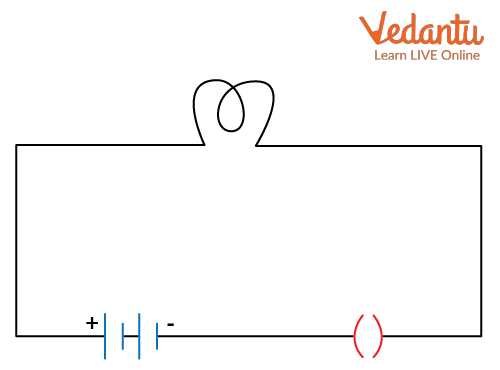Open Circuit

### 2. Closed Circuit

The flow of electrons in a closed circuit is from the negative terminal to the positive terminal.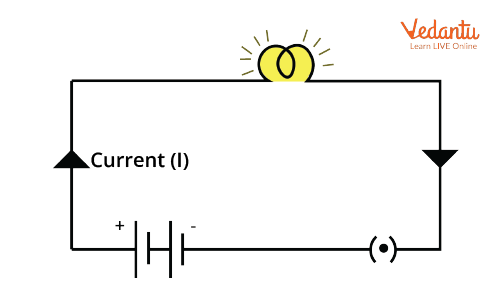Closed Circuit

### 3. DC Circuit

When the circuit has a DC source and the current value does not change periodically, the circuit is a DC circuit.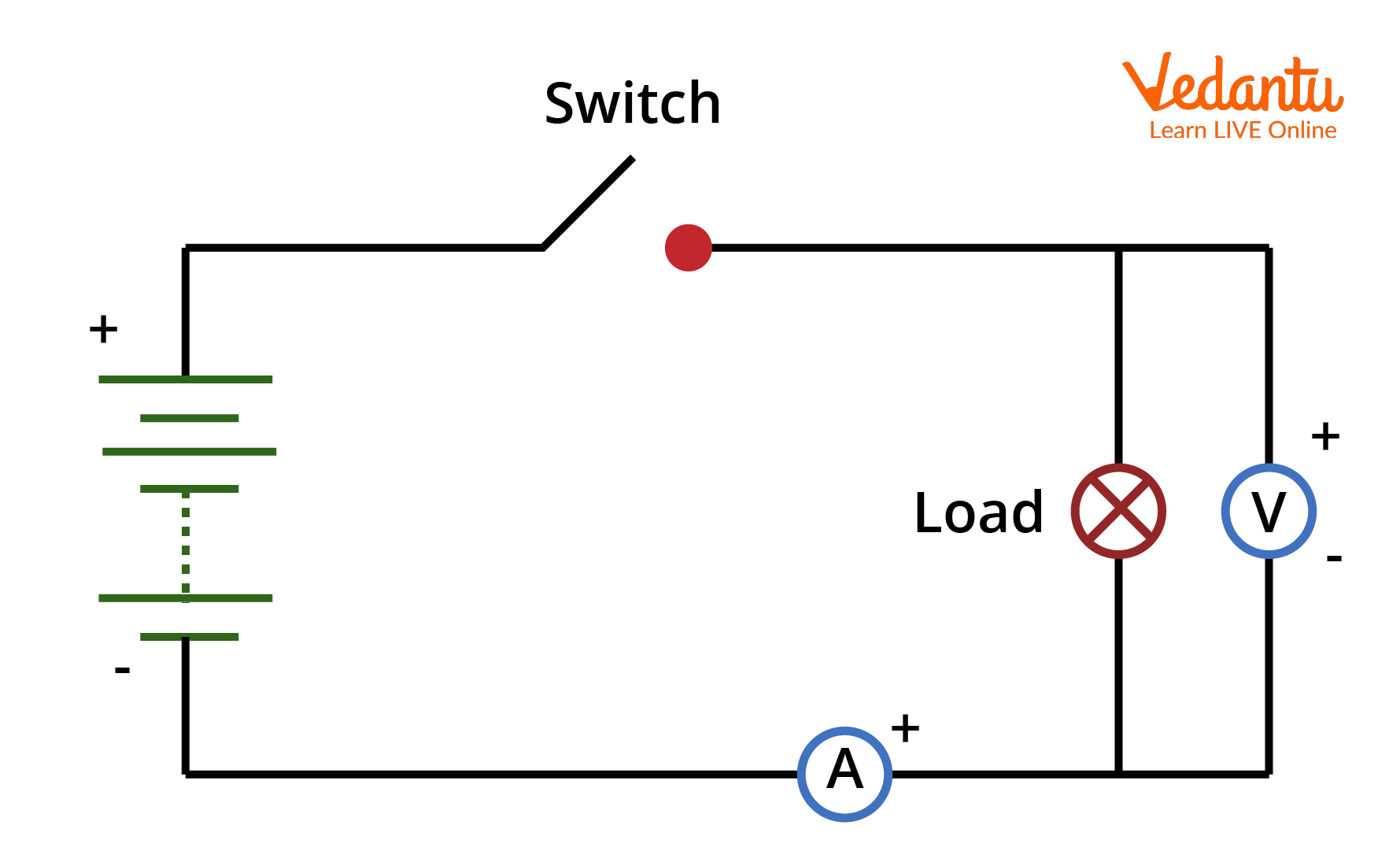DC Circuit

### 4. AC Circuit

In an AC circuit, the circuit has an AC source and the value of the current changes periodically.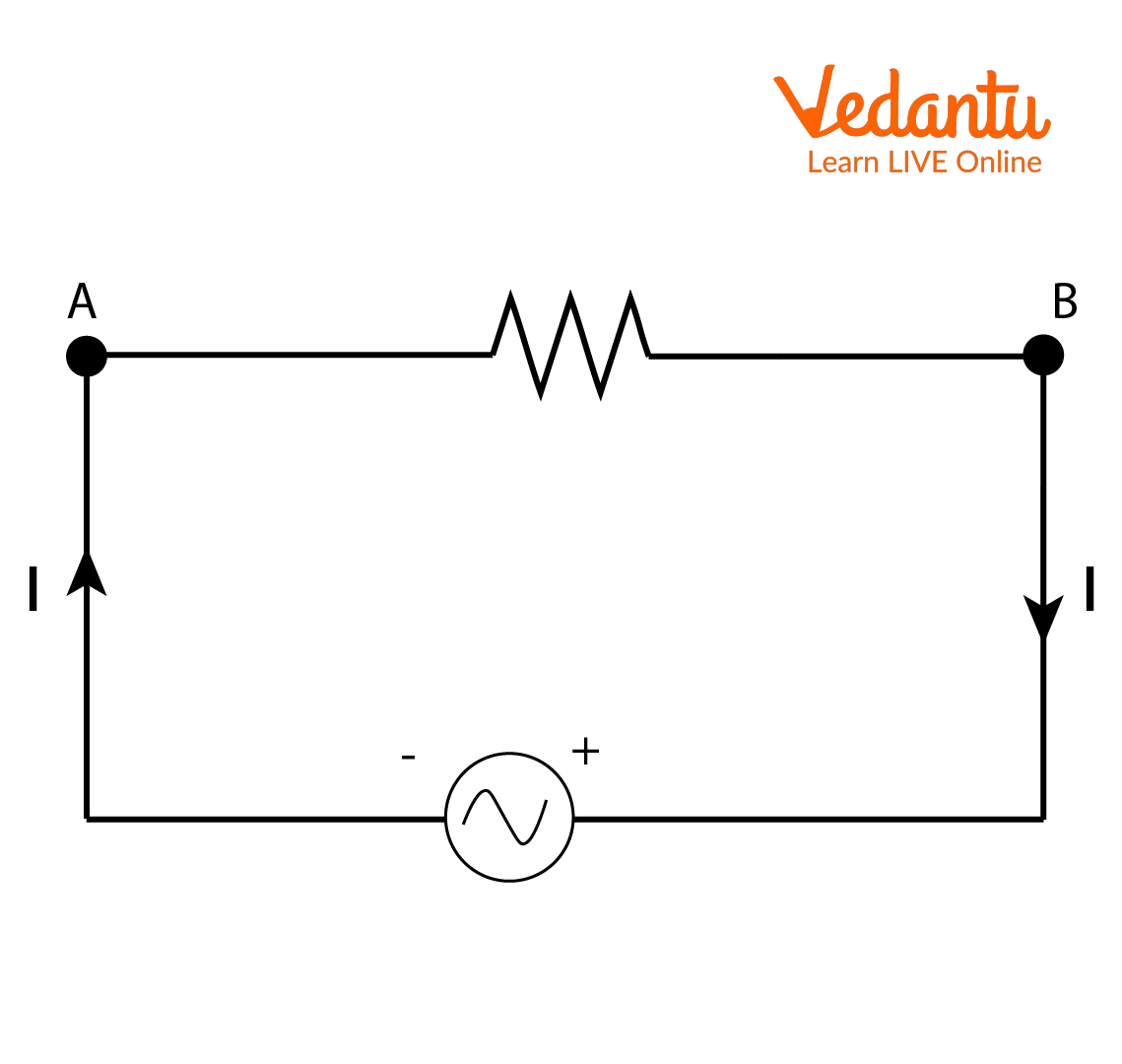AC circuit

### 5. Series Circuit

In a series circuit, the resistors are connected in series. The circuit is also termed as end-to-end connection or cascade connection. In a series circuit, the amount of current flowing in all resistors is the same.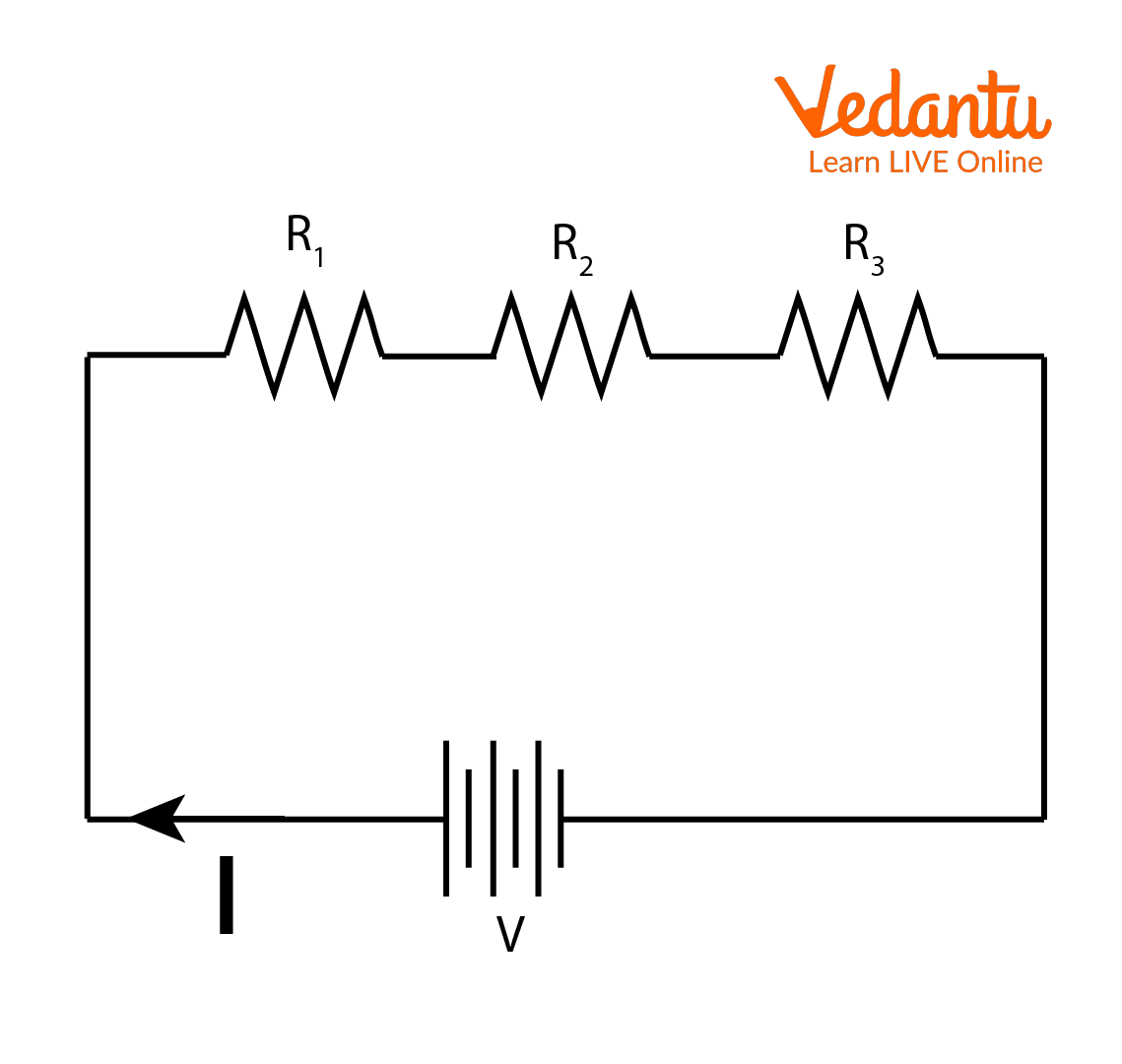Series Resistance Circuit

The equivalent resistance connected in series is denoted as Rseries=R1+R2+R3.

### 5. Parallel Circuit

When the resistors in circuit are connected in parallel or shunt, the circuit is called a parallel circuit. In a parallel circuit, the total currents divide as per the resistance in each branch.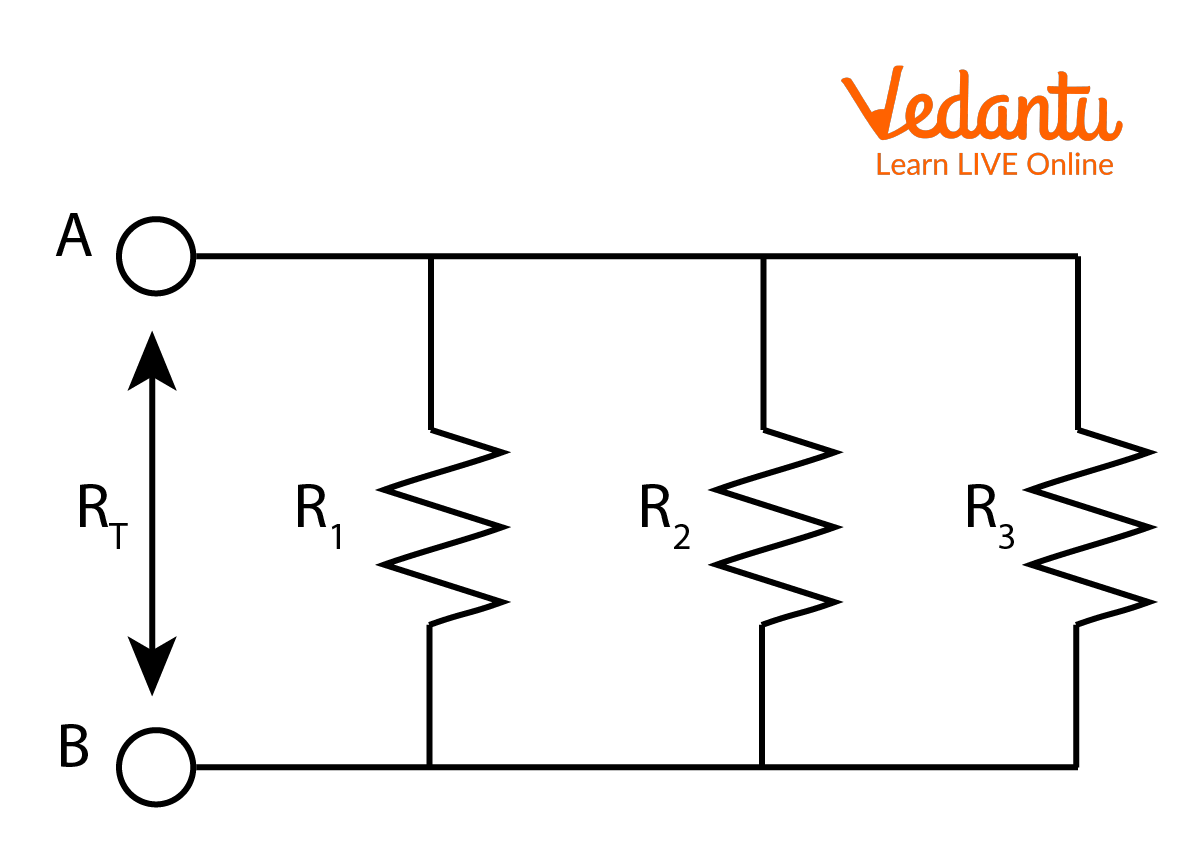Resistors in Parallel Combination

The equivalent resistors in this case are calculated as $\dfrac{1}{R_{e q}}=\dfrac{1}{R_{1}}+\dfrac{1}{R_{2}}+\dfrac{1}{R_{3}} \ldots+\dfrac{1}{R_{n}}$.

### Series-Parallel Circuit

A series-parallel circuit is the one in which the resistors are connected in series as well as parallel. In this circuit, different values of voltage are expected.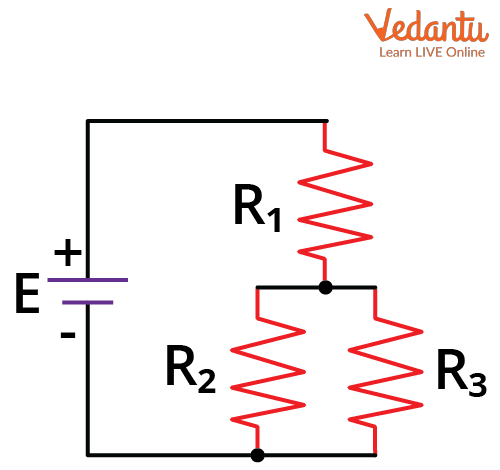Series-Parallel Circuit

## Circuit Solving Methods

Circuit solving techniques are required to simplify the complex circuit diagrams. These circuit solving techniques include:

1. Ohm's Law

2. Kirchhoff's Laws (KVL and KCL)

3. Superposition Theorem

### 1. Ohm's Law

The Ohm’s law states that the potential difference between the ends of the conductor is directly proportional to the current flowing through it.

Mathematically, Ohm's law is denoted as:

$V \propto I$

V=IR

where R is the constant of proportionality and the resistance of the conductor.

The SI unit of resistance (R) is Ohms and is denoted as $\Omega$. The resistance also depends on the dimensions of the conductor.

### 2. Kirchhoff's Laws (KVL and KCL)

An electric circuit can be complex, especially when a number of resistors are connected in series and parallel. In such cases, the resistance value for series and parallel derived above might not be sufficient. Thus, to simplify the circuit, we use Krichoff’s laws, which are the Krichoff’s current and voltage law. Now, let us understand each of these laws in detail.

### 3. Kirchhoff's Current Law (KCL) or Junction Rule

It states that at a junction, the sum of  the incoming currents and the outgoing currents is equal.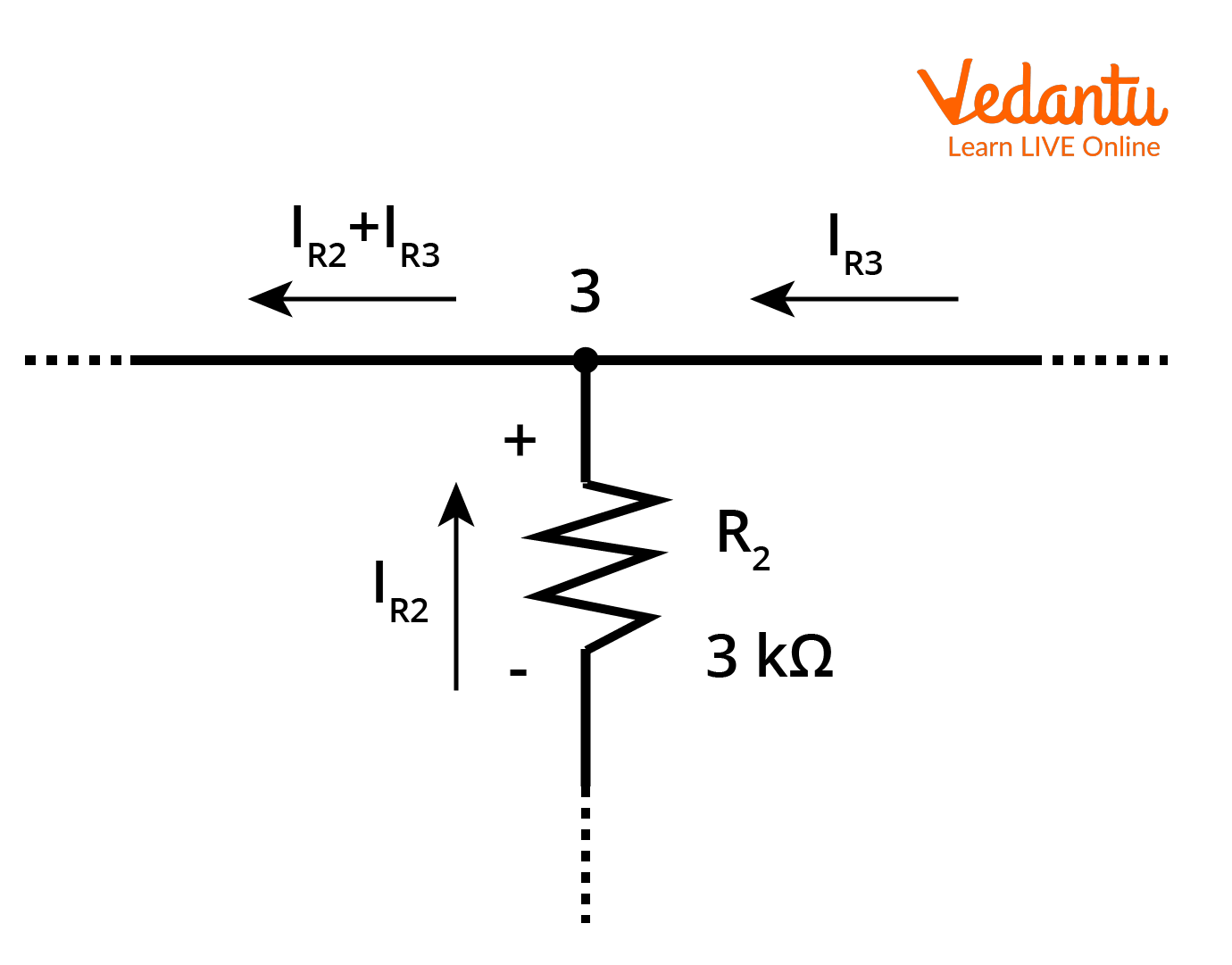Kirchoff’s Current Law

### 4. Kirchhoff's Voltage Law (KVL) or Loop Rule

It states that the sum of voltage drops or potential in a closed loop is equal to zero.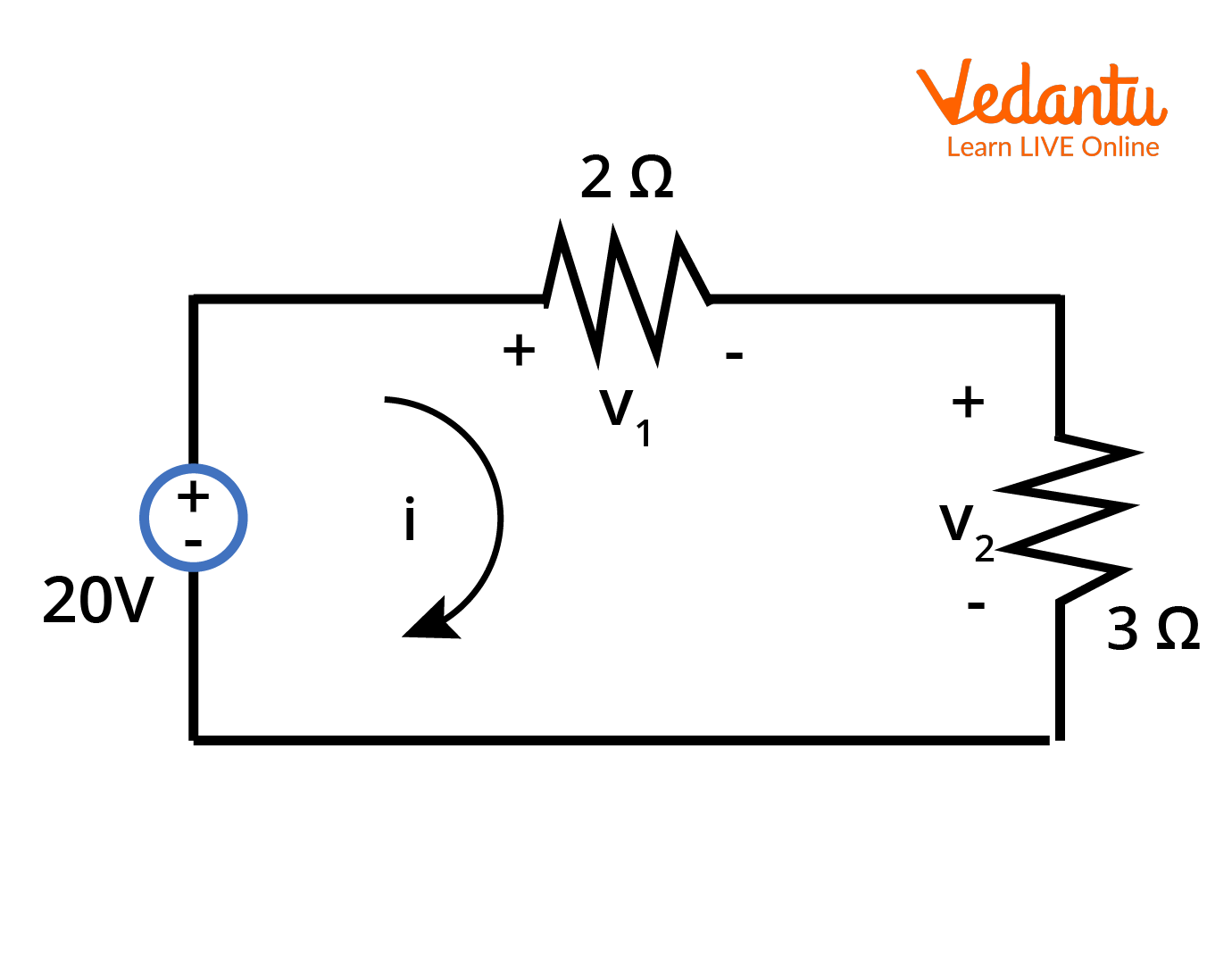Kirchhoff's Voltage Law

### 5. Superposition Theorem

It is based on the concept of linearity between excitation and response in an electric circuit. If multiple sources in an electric circuit are acting at the same time, the response in a particular branch is equal to the sum of the responses calculated by taking one source at a time.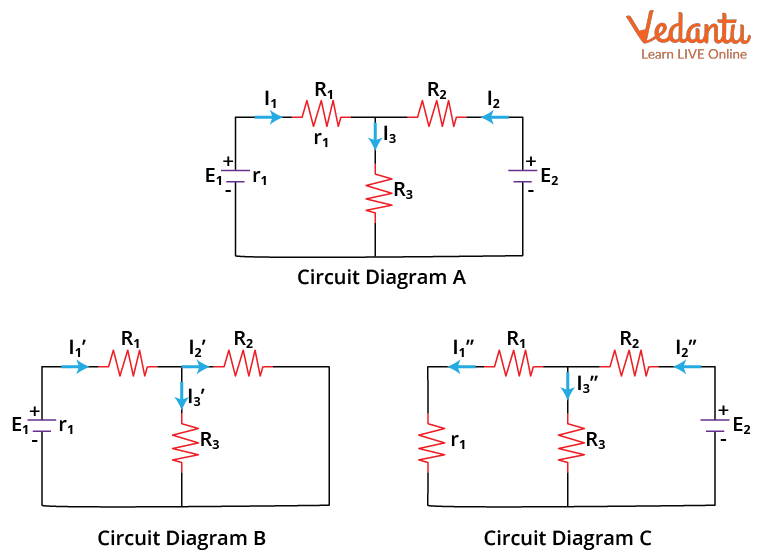Visual Representation of Superposition Theorem

Superposition theorem is fundamental when we need to find whether a circuit is linear or nonlinear. To deduce that a circuit is linear, it should be additive and homogeneous. Voltage and current follow the superposition theorem but not power. Also, the superposition theorem comes in handy when several voltage or current sources are connected in a circuit.

## How to Solve a Basic Electric Circuit Problem?

To solve a basic electric circuit problem, we need to follow the below steps:

• Identifying the Node Voltages

The ground node is identified and other nodes are marked as 1,2,3 depending on the circuits and how many nodes are there in a circuit. So, how do we identify a node in an electric circuit? A node is a point or a junction where two or more circuit elements meet.  Mathematically, in an electric circuit, there will be n-1 nodes.

• Next, Calculate the Equivalent Resistance

A circuit might constitute several resistors, and these may be connected in series or in parallel. As discussed above, series equivalent resistance is calculated as the sum of individual resistors whereas parallel equivalent resistors are a sum of the inverse of individual resistors.

• Now, We Calculate the Current

Using Ohm's law, we can find the total current in the circuit or in a particular branch. For a parallel circuit, currents can be calculated using the current divider rule.

The steps listed above are for a basic circuit. Circuits can be quite complex in real life.

## Electrical Circuit Theory Problems and Solutions

Electric circuit problems can be easily solved using the methods stated above, be it a simple or a complex circuit. Let us understand the application for each of these circuit solving techniques with some examples below:

Question 1: Three equal resistances are connected to a battery and consume a power of 100 watts. If the resistors are now connected in parallel, what will be the power consumed?

Solution:

As power is denoted as $P=\dfrac{V^{2}}{R}$, thus, when the resistors are connected in series, the power will be $P=\dfrac{V^{2}}{3R}$ or $\dfrac{V^{2}}{3R}=100$

$\Rightarrow \dfrac{V^{2}}{R}=300$

As the resistors are in parallel, the equivalent resistance will be $\dfrac{R}{5}$

The power consumed now will be $P=\dfrac{5V^{2}}{R}$

$\Rightarrow \dfrac{V^{2}}{R}=5 \times 300=1500$

Thus, the power consumed is 1500 watts.

Question 2: A uniform wire of length L and radius r has a resistance of 200 $\Omega$. If the wire is mouled as a wire of radius $\dfrac{r}{4}$, what will be the new resistance?

Solution:

Resistance of a wire of length L and radius r is given by

\begin{align} &R=\dfrac{\rho L}{A} \\ &\Rightarrow 200=\dfrac{\rho L}{A} \\ &\Rightarrow 200=\dfrac{\rho L}{\pi r^{2}} \ldots(1) \\ &R^{\prime}=\dfrac{\rho L}{A} \\ &\Rightarrow R^{\prime}=\dfrac{\rho L}{\pi(\dfrac{r }{ 2})^{2}} \\ &\Rightarrow R^{\prime}=\dfrac{4 \rho L}{\pi r^{2}} \ldots(2) \end{align}

Putting (1) in (2), we get

$\Rightarrow R^{\prime}=4(20 \Omega)=800 \Omega$

## Conclusion

Circuit solving is an important concept that one must understand thoroughly. Circuit solving entails numerous circuit solving methods like Ohm’s law, KVL, KCL, Superposition, Thevenin’s maximum power theorem, and so on. Electric circuits consist of passive elements like resistors, capacitors, inductors, and active elements like independent voltage and current sources. An electric circuit may also consist of dependent voltage and current sources. Thus, the current solving techniques mentioned above come in handy when solving such circuits.

Last updated date: 17th Sep 2023
Total views: 127.5k
Views today: 3.27k

## FAQs on Circuit Solving - Circuit Theory Problems and Solutions for JEE

1. Derive the equivalent capacitance when several capacitors are connected in series and parallel, respectively.

The equivalent value of capacitors connected in series and parallel is the inverse of how we calculate equivalent resistances for the same. N capacitors connected in parallel are denoted as: $C_{n}=C_{1}+C_{2}+\ldots+C_{n-1}$ whereas the capacitors connected in series are denoted as $\dfrac{1}{C_{n}}=\dfrac{1}{C_{1}}+\dfrac{1}{C_{2}}+\ldots+\dfrac{1}{C_{n-1}}$.

2. Derive the equivalent inductance when several inductors are connected in parallel and series, respectively.

The series and parallel inductors and their equivalent can be calculated in the same way as we calculate the equivalent resistance for resistors. The equivalent inductance for inductors connected in series is denoted as $L_{n}=L_{1}+L_{2}+\ldots+L_{n-1}$

Whereas inductors in parallel are calculated as $\dfrac{1}{L_{n}}=\dfrac{1}{L_{1}}+\dfrac{1}{L_{2}}+\ldots+\dfrac{1}{L_{n-1}}$.